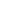# Free Arithmetic Progression Worksheets

Download free printable Arithmetic Progression Worksheets to practice. With thousands of questions available, you can generate as many Arithmetic Progression Worksheets as you want.

## Sample Arithmetic Progression Worksheet Questions

1.

The angles of a quadrilateral are in Ap with common difference 20°. what is the second least angle

1.

100

2.

60

3.

80

4.

120

2.

The sum of all natural numbers between 250 and 1000 which are divisible by 9

1.

52542

2.

52524

3.

54252

4.

54625

3.

For what value of k, are the numbers x, (2x + k) and (3x + 6) three consecutive terms of an A.P

1.

k=3

2.

k=4

3.

k=5

4.

k=6

4.

If  4/5, a , 2 are three consecutive terms of an A.P., then find the value of a

1.

6/5

2.

8/5

3.

5/6

4.

7/5

5.

The nth term of an AP is 3n + 5. what is the common difference

1.

5

2.

6

3.

3

4.

9

6.

The Sum of n terms of an A.P. is 5n2 – 3n. Hence find its 10th term

1.

376

2.

378

3.

374

4.

372

7.

The sum of 4th and 8th terms of an A.P. is 24, and the sum of 6th and 10th terms is 44. what is the first term.

1.

-13

2.

13

3.

15

4.

-15

8.

the sum of all three digit numbers which are divisible by 7 is

1.

73066

2.

70366

3.

70336

4.

73306

9.

Which term of the A.P.: 21, 18, 15, .... is zero

1.

7

2.

6

3.

8

4.

9

10.

If the sum of first 7 terms of an A.P. is 49 and that of first 17 terms is 289, find the sum of n terms.

1.

n^2

2.

n^3

3.

n/2

4.

2n

Worksheets by UrbanPro

Our worksheets are designed to help students explore various topics, practice skills and enrich their subject knowledge, to improve their academic performance. Designed by Experts who have extensive experience and expertise in teaching a subject, these worksheets will improve your child's problem-solving skills and subject knowledge in a fun and interactive manner.
Check out our free customized worksheets across school boards, grades, subjects and levels of subject knowledge. You can download, print and share these worksheets with anyone, anywhere, anytime!

Get a custom worksheet to practice!

Select your topic & see the magic.

subjectSelect Chapter(s)

Chapters & Subtopics